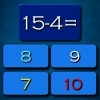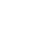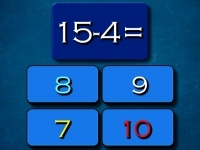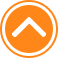×

PlaySubtraction Math Challenge72%Device: 💻 Pc   📱 Mobile
Rating: 72%
🎲Subtraction Math Challenge

Subtraction Math Challenge is fun math game suitable for all ages. I hope you like math and this game is just to test your math skills. Ten seconds to answer each quastion. Have fun playing. Play Subtraction Math Challenge online at kiz10.org. You can play it in fullscreen to have fun with it. Subtraction Math Challenge is a great Kiz10 game to play with no cost.

🎮 Instructions

Use Left Mouse button to Play

📂 Categories

puzzle, arcade, mathka, numbers, school, thinking, mathematical, mathematics, igrice, mathematic, math..

👌 Recommended
📌 Tags